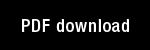Acquiring rule sets as a product of learning in a logical neural architecture

Author(s): Caudell, T.P. | Healy, M.J. |

Year: 1997

Citation: IEEE TRANSACTIONS ON NEURAL NETWORKS Volume: 8 Issue: 3 Pages: 461-474

Abstract: Envisioning neural networks as systems that learn rules calls forth the verification issues already being studied in knowledge-based systems engineering, and complicates these with neural-network concepts such as nonlinear dynamics and distributed memories, We show that the issues can be clarified and the learned rules visualized symbolically by formalizing the semantics of rule-learning in the mathematical language of two-valued predicate logic, We further show that this can, at least in some cases, be done with a fairly simple logical model, We illustrate this,vith a combination of two example neural-network architectures, LAPART, designed to learn rules as logical inferences from binary data patterns, and the stack interval network, which converts real-valued data into binary patterns that preserve the semantics of the ordering of real values, We discuss the significance of the formal model in facilitating the analysis of the underlying logic of rule-learning and numerical data representation, We provide examples to illustrate the formal model, with the combined stack interval/LAPART networks extracting rules from numerical data.

Topics: Machine Learning, Models: ART 1, Modified ART,